Learning Unit

 Page 1/4 Date 25.04.2017 Size 206.35 Kb. #17774
1   2   3   4

Learning Unit

Planning Guide

 Title Solving One- and Two-Step Equations Teacher / Team Algebra I Team Grade 8-9 Subject Algebra I

(What decisions do I need to make as I develop a Learning Unit?)

Decision 1: Concept Map of Learning Unit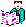Unit / Topic Concept

 Solving One- and Two-Step Equations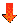Unit Summary – Paragraph Description

 The student will solve linear equations using addition, subtraction, multiplication and division. Students will express rational numbers in equivalent form, simplify numerical and algebraic expressions, evaluate simple algebraic expressions and formulas, and translate algebraic expressions and equations.

Major Concepts / Skills

What key knowledge and skills will students acquire as a result of this unit?

CRCT Domains/EOCT Domains - QCC Objectives

(List by heading (domain) followed by numbered QCC’s)

 QCC 1: Solve problems throughout this course that involve selecting appropriate approaches and tools, using estimating strategies to predict computational results, and judging reasonableness of results. QCC 3: Communicates mathematical ideas by using language and symbolism by reflecting upon and clarifies thinking about mathematical ideas and relationships, formulating mathematical definitions and expressing generalizations discovered throughout investigations, expressing mathematical ideas both orally and in wiring, interpreting written presentations of mathematics and asking clarifying and extending questions related to mathematics about which they have read or heard. QCC 8: Students will solve linear equations using a variety of methods such as manipulatives and technology. QCC 9: Students will solve problems involving linear equations. QCC 6: Students will distinguish between relations and functions, and identify the domain and range. CRCT Domain Algebraic Fundamentals 1.1:Students solve problems that involve selecting appropriate approaches and tools, using estimating strategies to predict computational results, or judging reasonableness of results CRCT Domain Algebraic Fundamentals 1.2: Students formulate mathematical definitions, interpret written presentations of mathematics, express generalizations, and express mathematical ideas in writing. CRCT Domain Solving Equations and Inequalities: Students solve word problems involving linear equations, inequalities, and systems of linear equations. CRCT Domain Functions and Their Graphs: Students solve problems by connecting patterns to the concept of functions; using patterns, relations, and functions to analyze, write, or sketch linear functions and their graphs; making a distinction between relations and function; and identifying the domain and range of a finite set of points.

Knowledge and Skills:

(Reference CRCT / End of Course Content Descriptors)

 Students will express rational numbers in equivalent form; simplify numerical and algebraic expressions, evaluate simple algebraic expressions and formulas, translate words into algebraic expressions and equations, represent problem situations with algebraic expressions and equations. Students will identify and apply properties of the real number system such as the Commutative, Associative, and Distributive Properties. Students will solve linear equations using a variety of methods.

Decision 2:

What are the Essential Questions of the Unit?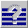Make sure there is at least one essential question for each major concept or skill. Most important essential questions also need extending / refining questions. All essential questions should be posted in the classroom.

 How do you determine the value of a variable in an equation? How are the signs, symbols, and words used in math? Why is PEMDAS important? How do you use numbers to represent words to write and solve an equation?

Decision 3:

What is the Performance / Product or Project that is the Culminating Activity of the Unit?

What evidence will show that students understand?

This is a summary description.

Describe in detail the product(s)/project(s).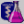The student will create a mathematical BINGO card with solutions from linear equations using addition, subtraction, multiplication and division. To formulate their equations solutions students will express rational numbers in equivalent form, simplify numerical and algebraic expressions, evaluate simple algebraic expressions and formulas, and translate algebraic expressions and equations.

Student Assignment Page for the Culminating Activity

Essential Question of the Culminating Activity:

What essential questions will guide this unit

and focus teaching and learning?

 Why is it important to use mental math in real life situations? How are opposite operations used to solve equations?

Paragraph Description of the Culminating Activity (including curriculum and unit goals)

 You have been hired by a book company to create a new type of mathematical BINGO. Your job is to develop a 5x5 BINGO card (attached) containing the solutions to one- and two-step equations of varying styles (i.e. 5x + 3 = 8; 8=3 + 5x)

Steps or Task Analysis of the Culminating Activity:

What student products/performances will provide evidence of desired learning?

By what criteria and scoring tools will student products/performances be evaluated?
 Student will design a 5 x 5 BINGO card with solutions from 32 one-and two-step equations they have created. Students will submit 32 equations which satisfy the following criteria: (4) 1 step equations using addition (4) 1 step equations using subtraction (4) 1 step equations using multiplication (4) 1 step equations using division (4) 2 step equations using multiplication and addition (4) 2 step equations using multiplication and subtraction (4) 2 step equations using division and addition (4) 2 step equations using division and subtraction The equations must : display a variety of operational styles (the variable must be in a variety of positions in the equations). Be neatly typed or written Contain 4 of each “type” of one-and two-step equations Contain neat and adequate work showing the solution circled or highlighted Have solutions represented on a 5 x5 BINGO card that is neat and legible, completely filled out (all 24 spaces are to be filled out in random with one free space), and the back of the BINGO card must be headed with class, date, and name.

Decision 4: Culminating Activity Rubric

for One-Step and Two-Step Equations

SCALE
CRITERIA

Equations

• Varied in operational style

• Neat

A variety of operational styles are apparent

Typed or written neatly

(32-24)

Only 2-3 styles are apparent.

Errors are present and mistakes are apparent

(17-9)

Only 1 style of equation is apparent.

Not neat or legible; many mistakes which effect the game

(8 or less)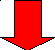Work

• Neat

Typed or written neatly

All equations have supporting work

YES

Errors are present and mistakes are apparent

Most equations have supporting work

Not neat or legible; many mistakes which effect the game

Few or no equations have supporting work

NO

Bingo Card

• Neat/legible

• Completely filled out

• Personalized

Typed or written neatly

YES

YES

Errors are present and mistakes are apparent

Not neat or legible; many mistakes which effect the game

NO

NO

 Letter Grade Number Grade Criteria A 100-95 Only 1 (#2) in any ONE section B 85 Any combination of #2’s and #3’s C 75 Only 1 (#1) in any section D 70 2-4 (#1’s) in any section F 50 5 or more #1’s in any section(s)

1. How will you help students know where they are headed and why (e.g. major assignments, performance tasks, and criteria which the work will be judged by)?

H- How will you hook students through engaging and thought provoking experiences (e.g., issues, problems and challenges) that point towards big ideas, essential questions and performance tasks?

1. What events, real or simulated, can students experience to make ideas and issues real? What learning activities will help students to explore the big ideas and essential questions? What instruction is needed to equip students for the final performances?

1. How will you cause students to reflect and rethink to dig deeper into the core ideas? How will you guide students in rehearsing, revising, and refining their work based on feedback and self-assessment?

1. How will students exhibit their understanding about their final performances and products? How will you guide them in self-evaluation to identify strengths and weaknesses in their work and set future goals?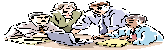Decision 5: Acquisition Lessons and Activities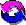(Hint: You must have at least one acquisition lesson for each essential question in your unit) (See Decision 2)

The 3 Phases of an Acquisition Lesson

Part 1- Beginning of the Lesson

2. Motivate Learner

3. Goal Setting with Essential Questions

Part 2Middle of the Lesson

1. Moving towards knowledge and Skills

1. Vocabulary

2. Declarative Content

3. Procedural Content

2. Collaborative Pairs in Distributed Summarizing and distributed guided practice

3. Re-teaching, Monitoring, Enrichment, Acceleration, Mastery Options

4. Formative Assessment is primary focus for Assessment

(GOAL = CONTINUOUS IMPROVEMENT)
Part 3End of the Lesson - Summarizing

1. Learner Summarizes, summarizes, summarizes

2. Assignments match learners’ preparation and learning level, NOT COVERAGE

3. Learners answer the overall unit Essential Question